Point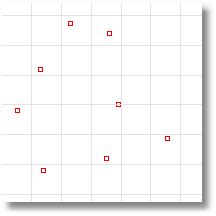Draws a single point object at a specified location.

Steps:

• Pick

a location for the point object.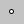Main2 > Point Point > Single PointCurve > Point Object > Single Point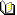Related topics…

Points

Draws multiple point objects.

Steps:

1. Pick locations for the point objects.

2. Press Enter

to end the command.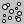Main2 > Multiple Points (Right click) Point > Multiple Points 3-D Digitizing > Multiple PointsCurve > Point Object > Multiple PointsRelated topics…

Divide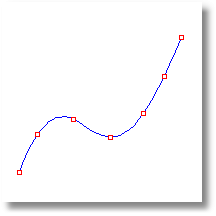Creates point objects by dividing a curve into a number of equal length segments or segments of a specified length.

Steps:

1. Select

curves.

2. Type the number of segments.

3. The point objects this command creates are spaced evenly along the curve, with an additional point at each end of the curve.

Notes

• To split the curve at the point objects created by the Divide command, use the command.

Options

Length

Creates point objects on a curve starting from the natural start of the curve. If you want points to start at the other end, use the command to flip the curve.

MarkEnds

Places a point at the ends of the curve.

### History enabled…

Changing the input objects changes the output objects.Point > Divide Curve by Number of Segments (Right click)Curve > Point Object > Divide Curve by > Number of SegmentsRelated topics…

PointGrid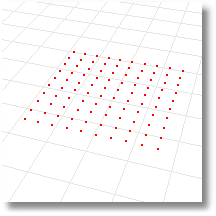Draws a rectangular grid of point objects from specified corner locations, two adjacent corners and a distance, perpendicular to the construction plane, or from a center.

Steps:

1. Type the number of points in the x-direction.

2. Type the number of points in the y-direction.

3. Pick a corner for the grid.

4. Pick for the other corner.

5. A point cloud
object is created with points placed in a rectangular array.

Options

Note: Point grids are drawn with the same options as the command.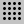Point > Point gridNoneRelated topics…

DrapePt

Creates a grid of point objects at the intersections of objects and points projected toward the construction plane in the current viewport.

Steps:

• Drag a rectangle over the objects to drape.

• A grid of points is created over the objects.

Options

See the Drape
command for option descriptions.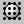Point > Drape Point Grid over ObjectsCurve > Point Object > Drape PointsRelated topics…

ClosestPt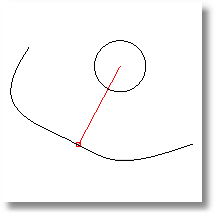Creates a point object at the closest point from a specified location to a selected object or on two objects where they are closest to each other.

Steps:

1. Select

the target objects.

2. Pick

the base point for the closest point calculation.

3. The distance is printed on the command line.

This command finds the location on an object you specify that is closest to the base point you previously picked, and it then creates a point object.

Options

Object

Point objects are created both of the selected objects at the points closest to each other.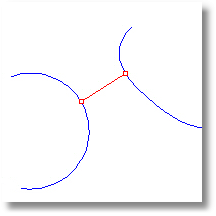Note: To select parts of objects, use sub-object selection
.

CreateLine

If Yes, a line is drawn between the base point and the closest point on the object.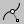Point > Closest PointCurve > Point Object > Closest PointRelated topics…

CrvEnd

Places a point object at the end of a curve.

Steps:

• Select

the curves.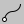Point > Mark Curve End (Right click)Curve > Point Object > Mark Curve EndRelated topics…

CrvStart

Places a point object at the start of a curve.

Steps:

• Select

the curves.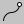Point > Mark Curve StartCurve > Point Object > Mark Curve StartRelated topics…

PointCloud

Creates a single object from selected point objects for selection and faster display.

Steps:

• Select

point objects and point clouds.

The point cloud object type improves Rhino’s performance when handling a large number of point objects imported from external files.

You can use the point cloud object to group any number of individual points into a single object, minimizing the amount of storage in the 3DM file and maintaining performance. You can snap and select points in the cloud as if they were just point objects. A point cloud is similar to a mesh object that does not display any wires between vertices.

To free all points in a point cloud use the command.

Options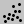Point > Point CloudCurve > Point Cloud > Create Point CloudRelated topics…